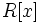# Category:Polynomial-closed properties of commutative unital rings

This category lists properties$p$ of commutative unital rings, such that if$R$ satisfies$p$, so does the polynomial ring$R[x]$.

The properties listed here need not be closed under the operation of taking the polynomial ring in infinitely many variables.

## Pages in category "Polynomial-closed properties of commutative unital rings"

The following 6 pages are in this category, out of 6 total.